EE 321 Lab
Lab 1: Amplifiers, Biasing, and AC Coupling
Prelab for Lab 1

Doing the prelab before coming to lab will get you out on time. Waiting until your lab session WILL KEEP YOU IN THE LAB WELL PAST DINNER.

The purpose of this lab is to measure the characteristics of an amplifier, and to use the characteristics to add a bias circuit at the input. We will represent the same amplifier three different ways. These are a block diagram, a model, and a schematic of the components. Figure 1 shows the block diagram of a generic amplifier. Figure 2 shows the model for a voltage amplifier. The characteristics which we need to measure are Ri, Ro and Avo.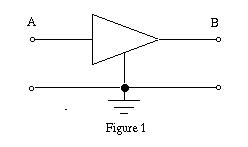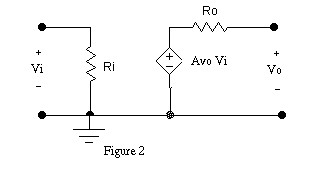We will use the circuit in Figure 3 as our amplifier. (Use a 741 or similar op-amp; the 741 pin out is shown in Figure 4.) In Figures 1, 2 and 3, the input to our amplifier is at A and the output at B. If we can find the three values Ri, Ro, and Avo we can use the model to accurately predict how the amplifier will work in a circuit.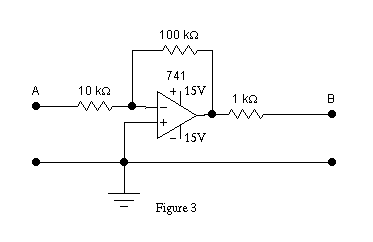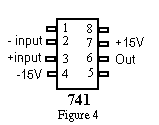• Build the amplifier.
1) Measure the transfer function, Avo, of the amplifier in the following manner.
• Connect the variable voltage source of Figure 5 to the input, and measure the voltage at both the input (A) and output (B) with a DVM. Plot the input voltage versus the output voltage. The gain, Avo, is the slope of this line. Compare this value to the one you found in the prelab.
•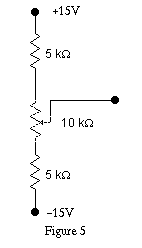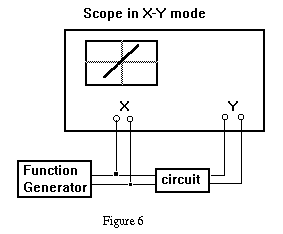• This process can be automated. Use the function generator and scope to trace the transfer function as shown in Figure 6. Store and copy the X-Y plot. Carefully mark the scales just as on the first graph.
• Compare your curve with the X-Y plot. What are the saturation voltages for the output?
2) Determine the input resistance Ri of the amplifier model circuit (that is the resistance from point A to ground).
• To do this add a test resistor between the variable source and the input (A).
• Make sure the output is NOT saturated.
• Measure the voltage of your variable source, and the voltage at point A.
• From a drawing of the input input circuit connected to the amplifier model you can see how Ri effects these voltages. Compute Ri.
• If the test resistor is not within a factor of 2 of Ri, change it to one about equal to Ri and make a more accurate determination of Ri. Why does this make the determination more accurate.
• Measure the test resistor with an ohm meter to determine its value accurately.
• Compute Ri again. Compare this value to the one you found in the prelab.
3) Determine the output resistance Ro.
• Using a short to measure isc may damage an actual amplifier, so in general, you should not short the output to try to measure Ro.
• Instead place a load resistance from B to ground, and note the decrease of the output voltage.
• To see how to analyze this, draw the output half of the amplifier model including the test resistor, marking measured voltages.
• Choose a test resistor of an appropriate size. (What is an appropriate size?)
• Compute Ro. Compare this value to the one you found in the prelab.
4) A capacitor will block DC voltages.

Use the circuit of figure 3.

• Input a 5 kHz, 1V p-p sine wave. Display and sketch the input and output.
• Use the function generator to add a 0.1 V offset to the input. What is the effect at the output?
• Add a 0.015 uF capacitor as shown in Figure 7 (Rs is the source resistance of the signal generator). How is the output affected.

•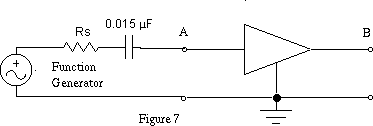• Why?
• Now, what effect does varying the input offset have on the output voltage?
5) Biasing the amplifier.
• Add the bias resistor R1 shown in Figure 8 to change the output operating point to about 5V

•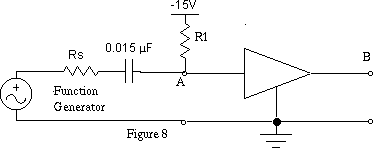• For an input vi of 0V the output vo should be 5.0V .
• The resistance values determined for the model can be used to calculate R1.
• With a sine input, sketch the output.
• What is the effect of the biasing on the output?